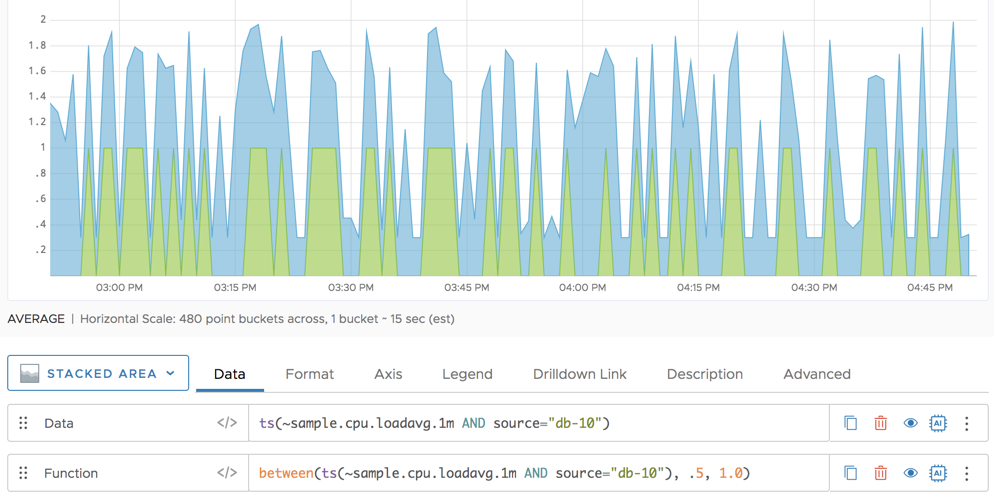Reference to the between() function

## Summary

``````between(<expression>, <lower>, <upper>)
``````

Returns 1 if `expression` is greater than or equal to `lower` and less than or equal to `upper`. Otherwise, returns 0. This function outputs continuous time series.

## Parameters

PropertyDescription
expression Expression to compare against an upper and lower limit.
lower Lower bound for the between() function.
upper Upper bound for the between() function.

## Description

The `between()` function determines whether the value of `expression` is greater than or equal to`lower` and less than or equal to `upper`. When the value of `expression` falls in between the values of `lower` and `upper`, the function displays 1 on the chart. When `expression` does not fall between `lower` and `upper`, the function displays 0 on the chart. The values for `expression`, `lower`, and `upper` can be any constant or ts() expression.

## Examples

The following example show the CPU load average per 1 minute as a blue line. The orange line shows a value of 1 where the metric is between .5 and 1.0, and shows a value of zero otherwise.Series Matching